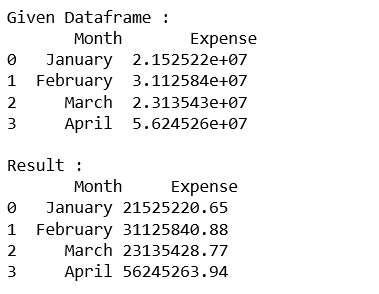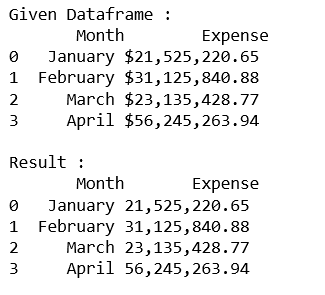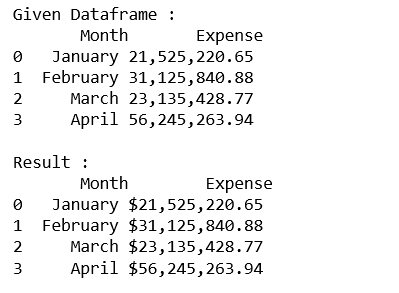Open in App
Not now

# Formatting float column of Dataframe in Pandas

• Difficulty Level : Easy
• Last Updated : 21 Aug, 2020

While presenting the data, showing the data in the required format is also an important and crucial part. Sometimes, the value is so big that we want to show only desired part of this or we can say in some desired format.

Let’s see different methods of formatting integer column of Dataframe in Pandas.

Code #1 : Round off the column values to two decimal places.

 `# import pandas lib as pd``import` `pandas as pd`` ` `# create the data dictionary``data ``=` `{``'Month'` `: [``'January'``, ``'February'``, ``'March'``, ``'April'``],``     ``'Expense'``: [ ``21525220.653``, ``31125840.875``, ``23135428.768``, ``56245263.942``]}`` ` `# create the dataframe``dataframe ``=` `pd.DataFrame(data, columns ``=` `[``'Month'``, ``'Expense'``])`` ` `print``(``"Given Dataframe :\n"``, dataframe)`` ` `# round to two decimal places in python pandas``pd.options.display.float_format ``=` `'{:.2f}'``.``format`` ` `print``(``'\nResult :\n'``, dataframe)`

Output:Code #2 : Format ‘Expense’ column with commas and round off to two decimal places.

 `# import pandas lib as pd``import` `pandas as pd`` ` `# create the data dictionary``data ``=` `{``'Month'` `: [``'January'``, ``'February'``, ``'March'``, ``'April'``],``        ``'Expense'``:[ ``21525220.653``, ``31125840.875``, ``23135428.768``, ``56245263.942``]}`` ` `# create the dataframe``dataframe ``=` `pd.DataFrame(data, columns ``=` `[``'Month'``, ``'Expense'``])`` ` `print``(``"Given Dataframe :\n"``, dataframe)`` ` `# Format with commas and round off to two decimal places in pandas``pd.options.display.float_format ``=` `'{:, .2f}'``.``format`` ` `print``(``'\nResult :\n'``, dataframe)`

Output:Code #3 : Format ‘Expense’ column with commas and Dollar sign with two decimal places.

 `# import pandas lib as pd``import` `pandas as pd`` ` `# create the data dictionary``data ``=` `{``'Month'` `: [``'January'``, ``'February'``, ``'March'``, ``'April'``],``        ``'Expense'``:[ ``21525220.653``, ``31125840.875``, ``23135428.768``, ``56245263.942``]}`` ` `# create the dataframe``dataframe ``=` `pd.DataFrame(data, columns ``=` `[``'Month'``, ``'Expense'``])`` ` `print``(``"Given Dataframe :\n"``, dataframe)`` ` `# Format with dollars, commas and round off``# to two decimal places in pandas``pd.options.display.float_format ``=` `'\${:, .2f}'``.``format`` ` `print``(``'\nResult :\n'``, dataframe)`

Output:My Personal Notes arrow_drop_up